Students can download Maths Chapter 5 Coordinate Geometry Unit Exercise 5 Questions and Answers, Notes, Samacheer Kalvi 10th Maths Guide Pdf helps you to revise the complete Tamilnadu State Board New Syllabus, helps students complete homework assignments and to score high marks in board exams.

## Tamilnadu Samacheer Kalvi 10th Maths Solutions Chapter 5 Coordinate Geometry Unit Exercise 5

Question 1.
PQRS is a rectangle formed by joining the points P(- 1, – 1), Q(- 1, 4) , R(5, 4) and S (5, – 1). A, B, C and D are the mid-points of PQ, QR, RS and SP respectively. Is the quadrilateral ABCD a square, a rectangle or a rhombus? Justify your answer.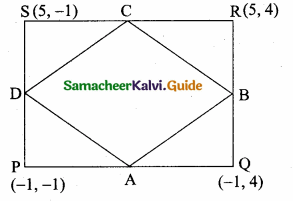Mid point of a line = ($$\frac{x_{1}+x_{2}}{2}, \frac{y_{1}+y_{2}}{2}$$)
Mid point of PQ (A) = ($$\frac { -1-1 }{ 2 }$$,$$\frac { -1+4 }{ 2 }$$)
= ($$\frac { -2 }{ 2 }$$,$$\frac { 3 }{ 2 }$$) = (-1,$$\frac { 3 }{ 2 }$$)
Mid point of QR (B) = ($$\frac { -1+5 }{ 2 }$$,$$\frac { 4+4 }{ 2 }$$) = ($$\frac { 4 }{ 2 }$$,$$\frac { 8 }{ 2 }$$) = (2,4)
Mid point of RS (C) = ($$\frac { 5+5 }{ 2 }$$,$$\frac { 4-1 }{ 2 }$$) = ($$\frac { 10 }{ 2 }$$,$$\frac { 3 }{ 2 }$$) = (5,$$\frac { 3 }{ 2 }$$)
Mid point of PS (D) = ($$\frac { 5-1 }{ 2 }$$,$$\frac { -1-1 }{ 2 }$$) = ($$\frac { 4 }{ 2 }$$,$$\frac { -2 }{ 2 }$$) = (2,-1)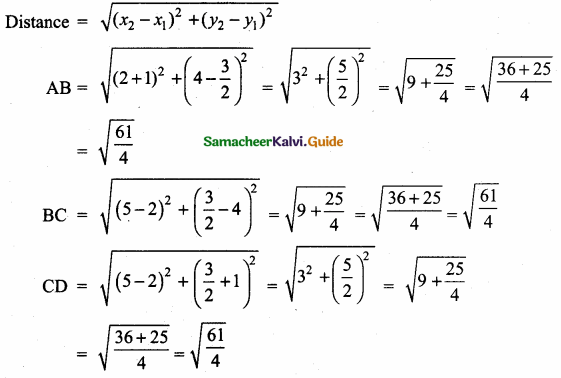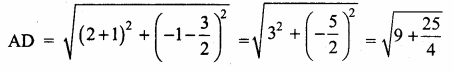img 355
AB = BC = CD = AD = $$\sqrt{\frac{61}{4}}$$
Since all the four sides are equal,
∴ ABCD is a rhombus.Question 2.
The area of a triangle is 5 sq. units. Two of its vertices are (2,1) and (3, -2). The third vertex is (x, y) where y = x + 3 . Find the coordinates of the third vertex.
Let the vertices A(2,1), B(3, – 2) and C(x, y)
Area of a triangle = 5 sq. unit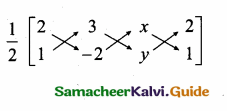$$\frac { 1 }{ 2 }$$ [x1y2 + x2y3 + x3y1 – (x2y1 + x3y2 + x1y3)] = 5
$$\frac { 1 }{ 2 }$$ [-4 + 3y + x – (3 – 2x + 2y)] = 5
-4 + 3y + x – 3 + 2x – 2y = 10
3x + y – 7 = 10
3x + y = 17 ……(1)
Given y = x + 3
Substitute the value ofy = x + 3 in (1)
3x + x + 3 = 17
4x = 17 – 3
4x = 14
x = $$\frac { 14 }{ 4 }$$ = $$\frac { 7 }{ 2 }$$
Substitute the value of x in y = x + 3
y = $$\frac { 7 }{ 2 }$$ + 3 ⇒ y = $$\frac { 7+6 }{ 2 }$$ = $$\frac { 13 }{ 2 }$$
∴ The coordinates of the third vertex is ($$\frac { 7 }{ 2 }$$,$$\frac { 13 }{ 2 }$$)Question 3.
Find the area of a triangle formed by the lines 3x + y – 2 = 0, 5x + 2y – 3 = 0 and 2x – y – 3 = 0
3x + y = 2 ……..(1)
5x + 2y = 3 ………(2)
2x – y = 3 ……….(3)
Solve (1) and (2) to get the vertices B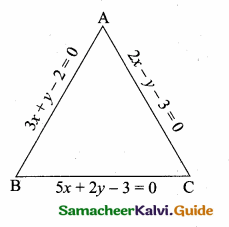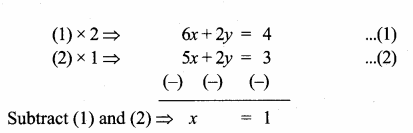Substitute the value of x = 1 in (1)
3(1) + y = 2
y = 2 – 3 = – 1
The point B is (1, – 1)
Solve (2) and (3) to get the vertices C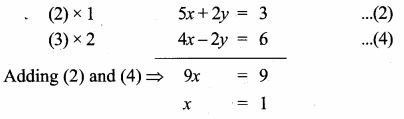Substitute the value of x = 1 in (3)
2(1) – y = 3 ⇒ -y = 3 – 2
– y = 1 ⇒ y = – 1
The point C is (1, – 1)
Solve (1) and (3) to get the vertices A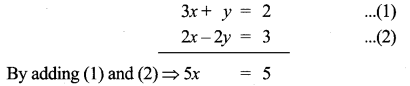Substitute the value of x = 1 in (1)
3(1) + y = 2
y = 2 – 3 = -1
The point A is (1, – 1)
The points A (1, – 1), B (1, -1), C(1, -1)
Area of ∆ABC = $$\frac { 1 }{ 2 }$$ [x1y2 + x2y3 + x3y1 – (x2y1 + x3y2 + x1y3)]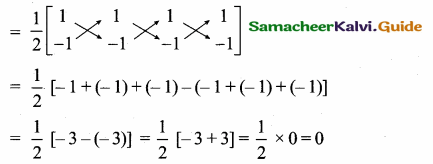Area of the triangle = 0 sq. units.
Note: All the three vertices are equal, all the point lies in a same points.Question 4.
If vertices of a quadrilateral are at A(- 5, 7), B(- 4, k), C(- 1, – 6) and D(4, 5) and its area is 72 sq.units. Find the value of k.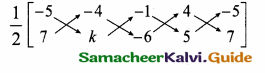Area of the quadrilateral ABCD = 72 sq. units.
$$\frac { 1 }{ 2 }$$ [(x1y2 + x2y3 + x3y4 + x4y1) – (x2y1 + x3y2 + x4y3 + x1y4)] = 72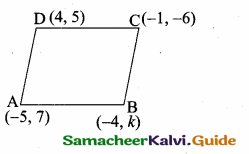-5k + 24 – 5 + 28 – (- 28 – K – 24 – 25) = 144
– 5k + 47 – k – 77 = 144
– 5k + 47 + k + 77 = 144
– 4k + 124 = 144
-4k = 144 – 124
– 4k = 20
k = -5
The value of k = – 5Question 5.
Without using distance formula, show that the points (-2,-1), (4,0), (3,3) and (-3,2) are vertices of a parallelogram.
The vertices A(-2, -1), B(4, 0), C(3, 3) and D(- 3, 2)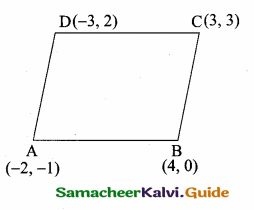Slope of a line = $$\frac{y_{2}-y_{1}}{x_{2}-x_{1}}$$
Slope of AB = $$\frac { 0+1 }{ 4+2 }$$ = $$\frac { 1 }{ 6 }$$
Slope of BC = $$\frac { 3-0 }{ 3-4 }$$ = $$\frac { 3 }{ -1 }$$ = -3
Slope of CD = $$\frac { 2-3 }{ -3-3 }$$ = $$\frac { -1 }{ -6 }$$ = $$\frac { 1 }{ 6 }$$
Slope of AD = $$\frac { 2+1 }{ -3+2 }$$ = $$\frac { 3 }{ -1 }$$ = -3
Slope of AB = Slope of CD = $$\frac { 1 }{ 6 }$$
∴ AB || CD ……(1)
Slope of BC = Slope of AD = -3
From (1) and (2) we get ABCD is a parallelogram.Question 6.
Find the equations of the lines, whose sum and product of intercepts are 1 and – 6 respectively.
Let the “x” intercept be “a”
y intercept = 1 – a (sum of the intercept is 1)
Product of the intercept = – 6
a (1 – a) = – 6 ⇒ a – a2 = – 6
– a2 + a + 6 = 0 ⇒ a2 – a – 6 = 0
(a – 3) (a + 2) = 0 ⇒ a – 3 = 0 (or) a + 2 = 0
a = 3 (or) a = -2
When a = 3
x – intercept = 3
y – intercept = 1 – 3 = – 2
Equation of a line is
$$\frac { x }{ a }$$ + $$\frac { y }{ b }$$ = 1
$$\frac { x }{ 3 }$$ + $$\frac { y }{ -2 }$$ = 1
$$\frac { x }{ 3 }$$ – $$\frac { y }{ 2 }$$ = 1
2x – 3y = 6
2x – 3y – 6 = 0

When a =-2
x – intercept = -2
y – intercept = 1 – (- 2) = 1 + 2 = 3
Equation of a line is
$$\frac { x }{ a }$$ + $$\frac { y }{ b }$$ = 1
$$\frac { x }{ -2 }$$ + $$\frac { y }{ 3 }$$ = 1
– $$\frac { x }{ 2 }$$ + $$\frac { y }{ 3 }$$ = 1
– 3x + 2y = 6
3x – 2y + 6 = 0Question 7.
The owner of a milk store finds that, he can sell 980 litres of milk each week at ₹ 14/litre and 1220 litres of milk each week at ₹ 16/litre. Assuming a linear relationship between selling price and demand, how many litres could he sell weekly at ₹ 17/litre?
Let the selling price of a milk be “x”
Let the demand be “y”
We have to find the linear equation connecting them
Two points on the line are (14, 980) and (16,1220)
Slope of the line = $$\frac{y_{2}-y_{1}}{x_{2}-x_{1}}$$
= $$\frac { 1220-980 }{ 16-14 }$$ = $$\frac { 240 }{ 2 }$$ = 120
Equation of the line is y – y1 = m (x – x1)
y – 980 = 120 (x – 14) ⇒ y – 980 = 120 x – 1680
-120 x + y = -1680 + 980 ⇒ -120 x + y = -700 ⇒ 120 x – y = 700
Given the value of x = 17
120(17) – y = 700
-y = 700 – 2040 ⇒ – y = – 1340
y = 1340
The demand is 1340 litersQuestion 8.
Find the image of the point (3,8) with respect to the line x + 3y = 7 assuming the line to be a plane mirror.
Let the image of P(3, 8) and P’ (a, b)
Let the point of intersection be O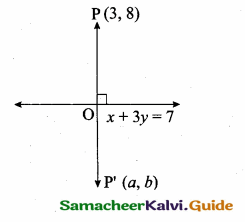Slope of x + 3y = 7 is – $$\frac { 1 }{ 3 }$$
Slope of PP’ = 3 (perpendicular)
Equation of PP’ is
y – y1 = m(x – x1)
y – 8 = 3 (x – 3)
y – 8 = 3x – 9
-8 + 9 = 3x – y
∴ 3x – y = 1 ………(1)
The two line meet at 0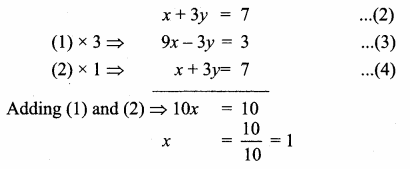Substitute the value of x = 1 in (1)
3 – y = 1
3 – 1 = y
2 = y
The point O is (1,2)
Mid point of pp’ = ($$\frac{x_{1}+x_{2}}{2}, \frac{y_{1}+y_{2}}{2}$$)
(1,2) = ($$\frac { 3+a }{ 2 }$$,$$\frac { 8+b }{ 2 }$$)
∴ $$\frac { 3+a }{ 2 }$$ = 1 ⇒ 3 + a = 2
a = 2 – 3 = -1
$$\frac { 8+b }{ 2 }$$ = 2
8 + b = 4
b = 4 – 8 = – 4
The point P’ is (-1, -4)Question 9.
Find the equation of a line passing through the point of intersection of the lines 4x + 7y – 3 = O and 2x – 3y + 1 = 0 that has equal intercepts on the axes.
Given lines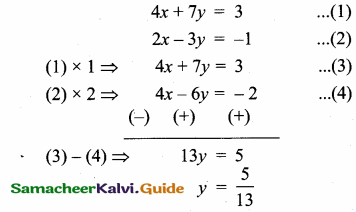Substitute the value of y = $$\frac { 5 }{ 13 }$$ in (2)
2x – 3 × $$\frac { 5 }{ 13 }$$ = -1
2x – $$\frac { 15 }{ 13 }$$ = -1
26x – 15 = -13
26x = -13 + 15
26x = 2
x = $$\frac { 2 }{ 26 }$$ = $$\frac { 1 }{ 13 }$$
The point of intersection is ($$\frac { 1 }{ 13 }$$,$$\frac { 5 }{ 13 }$$)Let the x – intercept and y intercept be “a”
Equation of a line is
$$\frac { x }{ a }$$ + $$\frac { y }{ b }$$ = 1
$$\frac { x }{ a }$$ + $$\frac { y }{ a }$$ = 1 (equal intercepts)
It passes through ($$\frac { 1 }{ 13 }$$,$$\frac { 5 }{ 13 }$$)
$$\frac { 1 }{ 13a }$$ + $$\frac { 5 }{ 13a }$$ = 1
$$\frac { 1+5 }{ 13a }$$ = 1
13a = 6
a = $$\frac { 6 }{ 13 }$$
The equation of the line is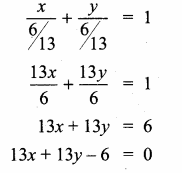Question 10.
A person standing at a junction (crossing) of two straight paths represented by the equations 2x – 3y + 4 = 0 and 3x + 4y – 5 = 0 seek to reach the path whose equation is 6x – 7y + 8 = 0 in the least time. Find the equation of the path that he should follow.
Two straight path will intersect at one point.
Solving this equations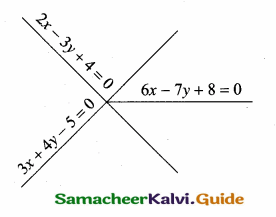2x – 3y + 4 = 0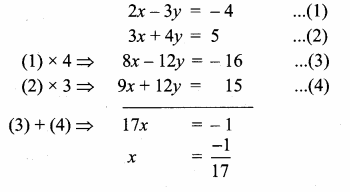Substitute the value of x = $$\frac { -1 }{ 17 }$$ in (2)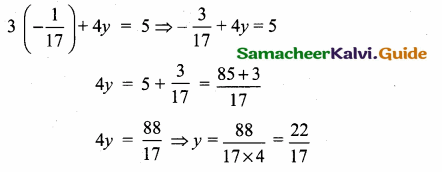The point of intersection is (-$$\frac { 1 }{ 17 }$$,$$\frac { 22 }{ 17 }$$)
Any equation perpendicular to 6x – 7y + 8 = 0 is 7x + 6y + k = 0
It passes through (-$$\frac { 1 }{ 17 }$$,$$\frac { 22 }{ 17 }$$)
7(-$$\frac { 1 }{ 17 }$$) + 6 ($$\frac { 22 }{ 17 }$$) + k = 0
Multiply by 17
-7 + 6 (22) + 17k = 0
-7 + 132 + 17k = 0
17k = -125 ⇒ k = – $$\frac { 125 }{ 17 }$$
The equation of a line is 7x + 6y – $$\frac { 125 }{ 17 }$$ = 0
119x + 102y – 125 = 0
∴ Equation of the path is 119x + 102y – 125 = 0